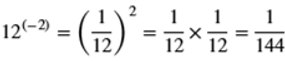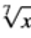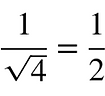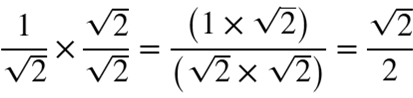#### 1.2 Powers and Roots

Powers and roots are very useful tools used by mathematicians and physicists to deal with large numbers and complex equations by compacting them. This makes calculations so much easier and neater. In this section we will discuss how to carry out calculations using powers and roots.

## Powers a.k.a Indices

Powers or indices or exponenents are a simple ways of expressing huge numbers. For example what is 429981696 divided by 2985984? Doing this calculation using a calculator will take up a bit time. However, if it was expressed in indices the calculation could be done in an instant:Expressing numbers in this form requires two componenent. A base and an index (power or exponent). The base is the number that will be repeatedly multiplied by itseself. The index is how many times the base has to be multiplied by it self.

###### Base###### Index / Power / Exponent

The base is 12 and index 8. Therefore, 12 has to be multiplied by itself for 8 times.

12 x 12 x 12 x 12 x 12 x 12 x 12 x 12 = 429981696

Important thing to note is that raising any number to a power zero is equal to 1:Now let's look at how to multiply and divide indices. When multiplying indices, if the bases are the same, then the index numbers can be added together. When dividing indices, if the bases are the same, the index numbers can be subtracted from each other. You must make sure that the bases are the same before doing this:You have to be extra careful when dividing because the way you substract the numbers will give you different values:But how do you deal with a negative index. A negative value indicates that the base value has to be changed to a reciprocal. By changing it to a reciprocal, you can get rid of the negative sign:Let's look at another example but this time with negative indices:It is important to note that when you raise the numbers within a bracket to a power then the indices of all those numbers will have to multiplied by the index outside the bracket:We discussed earlier that the above simplications can only be done if the bases are equal. What can we do if they are not? Well you will have to check and see if you can manipulate the equation to make the bases equal. If you can't then you cannot simplify it any further. Let's look at an example:The bases in this case are different. However, 2 is a factor of 10; therefore, you could rewrite 10 as 2 x 5. This is shown below:It can no longer be simplified because both 2 and 5 are prime numbers. Therefore, it can be left as it is or solved to get a final value.

## Roots

The root of a number, say x, is a number that when multiplied by itself a set number of times will give you that number x. A square root is a number that is multiplied by itself twice to give x. A cubic root is a number multiplied by itself three times to give you x. 4th root is a number multiplied by itself 4 times to give you x and so on.

For example, what is the square root of 9. Since 3 x 3 = 9, the square root is 3. Now what is the cubic root of 9? This is not straight forward and you will need a calculator to get a quick answer. Cubic root of 64 is 4. 4th root of 16 is 2.Let's look at how to write and express a root in an equation. The notation used to express a root is called the radical sign or radix. The number, whose root is being calculated is placed inside the open box. The type of root is placed outside the box. Let's say you looking for the square root, cubic root and 7th root of a number x. This is how to express them:The square root is usually expressed by using the radix without a 2 but there is nothing wrong with putting the two.A root can also be expressed using an index. When expressing a number squared, 2 is written in superscript next to the number. When expressing a square root of a number, the reciprocal of 2 is written in superscript next to the number:The square root of x ^2 is:##### Surds

Sometimes calculating the root of number will give you an answer that when expressed in decimal form keeps going on forever.

For example the square root of 2 is 1.41421356237309504880168872420969807856967187537694............

It just keeps on going. In these cases where the root of a number cannot be simplified to a finite decimal number, it is left in its root form. These numbers are called surds.

Another example of a surd is square root of 3 = 1.7320508075688772............

##### Rationalizing Denominators

Sometimes having an unsolvable root in a denominator can complicate things. These are known as iirational denominators. Rationalizing such denominators by taking away the roots from the denominator to the numerator will calculation a lot easier. Let's look at some simple examples:Having square root 4 in the denominator is no problem because the root can be solved and gotten rid of. But having square root 2 in the denominator would mean that the root cannot be removed since it is a surd.We can make this number easier to work with by moving the irrational number to the numerator. To do that we will have to multiply both the numerator and denominator by square root 2 as shown below:By squaring the square root you can get rid of the square root. Let's a more difficult example:## Simplifying Powers and Roots

Now that we know how to deal with powers and roots, lets look at how we can simplify equations containing both of these. It this section we will just work through a couple examples.Try to understand how each and every step in the calculations were performed.